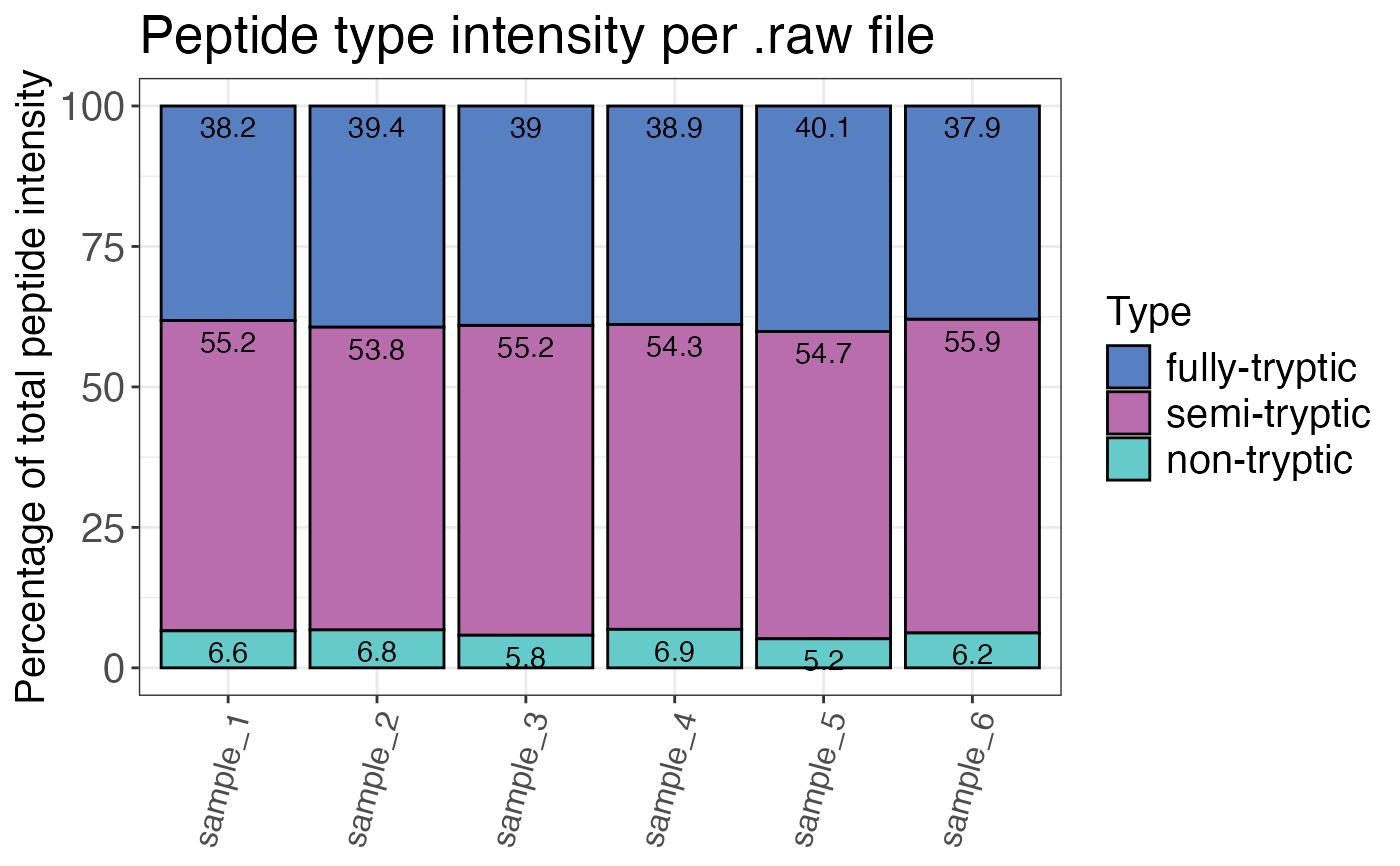Calculates the percentage share of each peptide types (fully-tryptic, semi-tryptic, non-tryptic) for each sample.

qc_peptide_type(
data,
sample,
peptide,
pep_type,
intensity,
remove_na_intensities = TRUE,
method = "count",
plot = FALSE,
interactive = FALSE
)

## Arguments

data a data frame that contains at least the input columns. a character column in the data data frame that contains the sample names. a character column in the data data frame that contains the peptide sequence. a character column in the data data frame that contains the peptide type. Can be obtained using the find_peptide and assign_peptide_type function together. a numeric column in the data data frame that contains the corresponding raw or normalised intensity values (not log2) for each peptide or precursor. Required when "intensity" is chosen as the method. a logical value that specifies if sample/peptide combinations with intensities that are NA (not quantified IDs) should be dropped from the data frame for analysis of peptide type distributions. Default is TRUE since we are usually interested in the peptide type distribution of quantifiable IDs. This is only relevant for method = "count". a character value that indicates the method used for evaluation. method = "intensity" calculates the peptide type percentage by intensity, whereas method = "count" calculates the percentage by peptide ID count. Default is method = count. a logical value that indicates whether the result should be plotted. a logical value that indicates whether the plot should be interactive.

## Value

A data frame that contains the calculated percentage shares of each peptide type per sample. The count column contains the number of peptides with a specific type. The peptide_type_percent column contains the percentage share of a specific peptide type.

## Examples

# Load libraries
library(dplyr)

set.seed(123) # Makes example reproducible

# Create example data
data <- create_synthetic_data(
n_proteins = 100,
frac_change = 0.05,
n_replicates = 3,
n_conditions = 2,
method = "effect_random"
) %>%
mutate(intensity_non_log2 = 2^peptide_intensity_missing)

# Determine peptide type percentages
qc_peptide_type(
data = data,
sample = sample,
peptide = peptide,
pep_type = pep_type,
intensity = intensity_non_log2,
method = "intensity",
plot = FALSE
)
#> # A tibble: 18 × 3
#>    sample   pep_type      peptide_type_percent
#>    <chr>    <fct>                        <dbl>
#>  1 sample_3 semi-tryptic                 55.2
#>  2 sample_4 semi-tryptic                 54.3
#>  3 sample_5 semi-tryptic                 54.7
#>  4 sample_6 semi-tryptic                 55.9
#>  5 sample_2 non-tryptic                   6.78
#>  6 sample_3 non-tryptic                   5.80
#>  7 sample_4 non-tryptic                   6.85
#>  8 sample_5 non-tryptic                   5.17
#>  9 sample_6 non-tryptic                   6.21
#> 10 sample_1 fully-tryptic                38.2
#> 11 sample_2 fully-tryptic                39.4
#> 12 sample_3 fully-tryptic                39.0
#> 13 sample_4 fully-tryptic                38.9
#> 14 sample_5 fully-tryptic                40.1
#> 15 sample_6 fully-tryptic                37.9
#> 16 sample_1 semi-tryptic                 55.2
#> 17 sample_2 semi-tryptic                 53.8
#> 18 sample_1 non-tryptic                   6.59
# Plot peptide type
qc_peptide_type(
data = data,
sample = sample,
peptide = peptide,
pep_type = pep_type,
intensity = intensity_non_log2,
method = "intensity",
plot = TRUE
)